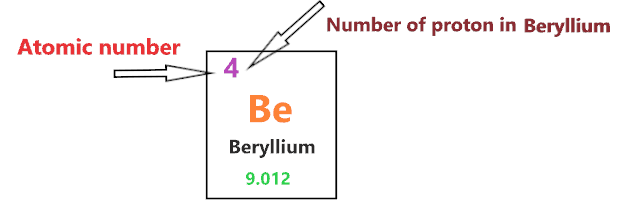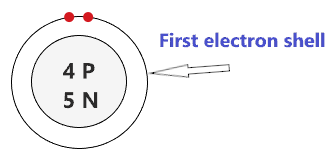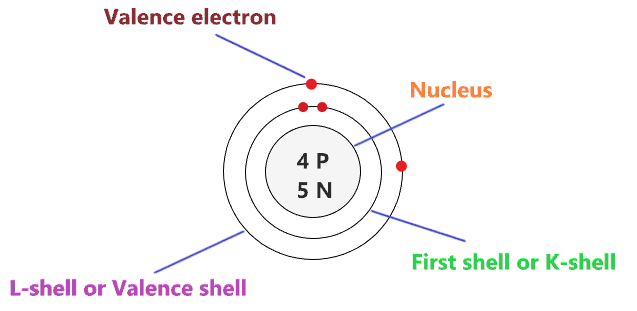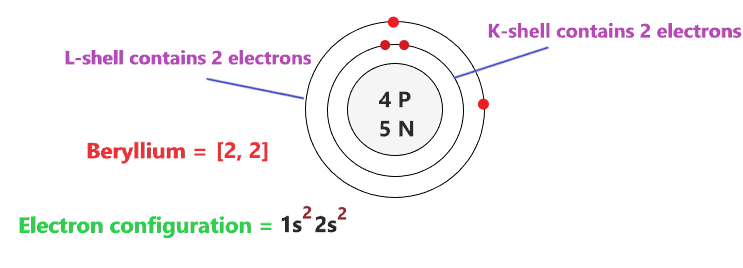# Bohr model of Beryllium atom - How to draw Beryllium(Be) Bohr-Rutherford diagram?

Home  > Chemistry Article > Beryllium Bohr model

The Bohr Model of Beryllium(Be) has a nucleus that contains 5 neutrons and 4 protons. This nucleus is surrounded by two-electron shells named K-shell and L-shell. The outermost shell in the Bohr diagram of Beryllium contains 2 electrons that also called valence electrons.

 Name Beryllium Bohr Model Number of neutrons 5 Number of protons 4 Number of electrons 4 Total electron shells 2 Electron in the First shell(K) 2 Electrons in the Second shell(L) 2 Total valence electrons in Beryllium 2
Page Contents

## How to draw Bohr Model of Beryllium(Be)?

Bohr model describes the visual representation of orbiting electrons around the small nucleus. It used different electron shells such as K, L, M, N…so on. These shells hold a specific number of electrons, the electron shell which is closest to the nucleus has less energy and the electron shell which is farthest from the nucleus has more energy.

Bohr diagram is very interesting and easy to draw. Here, we will draw the Bohr diagram of the Beryllium atom with some simple steps.

## Steps to draw the Bohr Model of Beryllium atom

1. Find the number of protons, electrons, and neutrons in the Beryllium atom

Protons are the positively charged particles and neutrons are the uncharged particles, both these are constituents of the atom nuclei. Electrons are the negatively charged particles that orbit the nucleus of an atom

To find the number of protons an atom contains, just look at their atomic number.

If the atomic number of atom is 8, then proton will also be 8, if atomic number is 9, then proton will also be 9.

So, the atomic number for Beryllium is 4, hence, the number of protons in the Beryllium atom is also 4.Now, to determine the number of neutrons in an atom, use this formula.

⇒ Number of neutrons in atom = Atomic mass of the atom(rounded to the nearest whole number) – Number of proton in an atom

For example, An atom have 12.977 atomic mass and 7 protons.

Then, to find the number of neutron, round the atomic mass to the near whole number, so, atomic mass 12.977 round to 13.

= (13 – 7 protons) = 6 number of neutrons in the atom

Now, To get the number of neutrons in a Beryllium atom, look at its atomic mass which is 9.012 rounded to 9, and the number of protons in Beryllium is 4.

∴ Hence, the number of neutrons in Beryllium atom = (9 – 4) = 5It should be noted that “The number of electrons in a neutral atom is equal to the number of protons”.

So, the Beryllium atom is neutral, hence, its number of electrons will be equal to the number of protons which is four as we already discussed.

⇒ The number of electrons in Beryllium atom = 4

⇒ The number of protons in Beryllium atom = 4

⇒ The number of neutrons in Beryllium atom = 5

2. Draw the nucleus of an atom

A nucleus is a dense and small region that contains the number of protons and neutrons of an atom.

In this step, we have to draw a small circle that consists of a number of protons and the number of neutrons of a Beryllium atom.3. Draw the First electron shell

“An electron shell may be thought of as an orbit followed by electrons around an atom’s nucleus.”

The first electron shell is also called the K-shell, this is the closest shell to the nucleus of an atom and can hold a maximum of two electrons.

As we know, Beryllium atoms have a total of 4 electrons. So, put two electrons from it, in the first shell, next to each other.We have successfully drawn the first shell of the Beryllium atom that can hold 2 electrons. As the Beryllium atom has a total of 4 electrons, and from 4 electrons we have used two electrons in the first shell.

∴ (4 – 2) = 2 electrons

Therefore, we are left with 2 electrons, let’s put them in the next shell of the Beryllium atom.

4. Draw the Second electron shell

The second shell also called the L-shell that can hold a maximum of 8 electrons. This shell is drawn after the first electron shell.

Since we already used two electrons in the first shell, now, we have to put the remaining 2 electrons in the second shell.

In the second electron shell, the electrons are added one at a time, starting from the top position then going in a clockwise direction.

In second shell, electrons are filled one at a time in clockwise direction as a clock position – 12 o’clock, 3 o’clock, 6 o’clock, 9 o’clock positions.So, we have to put 2 remaining electrons of the Beryllium atom in the second shell, hence, start from the top position, putting electrons one at a time, and go in a clockwise direction.### Bohr model of the Beryllium atom

That’s all, this is our Bohr model of the Beryllium atom that contains 4 protons and 5 neutrons in the nucleus region, and 4 electrons are orbited around the nucleus, two electrons in the first shell, and two electrons in the second shell.

## Find Valence electron of Beryllium through its Bohr diagram

Valence electrons are found in the outermost shell of an atom and they can take participate in the formation of a chemical bond. These electrons have more energy compare to the inner shell electrons.

From the Bohr diagram of an atom, we can easily find the number of valence electrons in an atom by looking at its outermost shell.

So, we have to find a valence electron in the Beryllium atom, for this, look at its Bohr diagram.

Bohr’s diagram of Beryllium has only two electron shells (K and L), the inner shell is K-shell and the outermost shell is L-shell.

Hence, the electrons found in the L-shell of the Beryllium atom are its valence electrons because it is the outermost shell that also called the valence shell.

The L-shell or outer shell of the Beryllium Bohr model contains 2 electrons, therefore, the number of valence electrons in the Beryllium atom is 2.## Electron dot diagram of the Beryllium atom

Electron dot diagram also called lewis structure which represents the valence electrons of atoms.

As, from the Bohr diagram of Beryllium, we got to know, it has 2 valence electrons.

So, just represent these 2 valence electrons around the Beryllium atom as a dot.## The electron configuration of Beryllium

Electron configuration is the distribution of electrons of an atom or molecule in atomic or molecule orbitals”

Beryllium has an atomic number of 4 and it contains a total number of 4 electrons. From the Bohr model of Beryllium, we know, 2 electrons are in the K-shell and 2 electrons are in the L-shell.

So, based on the shell, the electronic configuration of the Beryllium atom is [2, 2].

Or the electronic configuration of Beryllium is 1s22s2 since it contains a total of 4 electrons, the first two-electron will go in 1s orbital and the next two in 2s orbital,

S orbital can hold maximum of 2 electrons.## FAQ

### How many electron shells a Beryllium Bohr model contains?

Electron shell also called energy level, you can find the number of electron shells for an element by knowing its period number in the periodic table.

The elements or atoms in the first period of the periodic table have one energy level or one electron shell, same as, the elements in the second period have two energy levels or two-electron shells and so on…

So, the Beryllium atom belongs to the 2nd Period in the periodic table, hence, the number of electron shells for the Bohr model of Beryllium will be 2 (K-shell and L-shell).

### What is the outer shell of the Bohr diagram of the Beryllium atom?

The outermost shell also called the valence shell, which contains valence electrons of the atom.

According to the Bohr diagram of Beryllium, the outer shell is L-shell which contains 2 valence electrons.

## Properties of Beryllium

1. It has a hexagonal closed packed crystal structure.’
2. It has a boiling point of 2469 °C and a melting point of 1287 °C.
3. It appears as white-gray metallic and lightweight in nature.
4. It is one of the rare elements in the universe.
5. The oxidation state of beryllium is +2.

## Summary

• The Bohr model of Beryllium is drawn with only two electron shells, the first shell contains 2 electrons and the second shell also contains 2 electrons.
• Beryllium is neutral and its atomic number is 4, hence, the number of protons and electrons available for its Bohr diagram is also 4.
• The number of neutrons for the Bohr diagram of Beryllium can be found by subtracting the number of protons from the atomic mass(rounded to the nearest whole).
• The electron configuration of Beryllium in terms of the shell is [2, 2], or in normal form, it is [He] 2s2.
##### Subscribe to Blog via Email

Join 2 other subscribers

Share it...